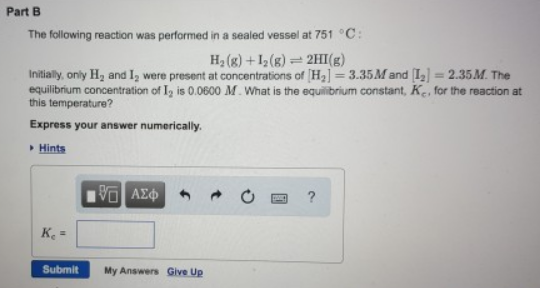# Problem: Part BThe following reaction was performed in a sealed vessel at 751°C: H2 (g) + I2 (g) ⇌ 2HI (g) Initially, only H2 and I2 were present at concentrations of [H2] = 3.35 M and [I2] = 2.35M. The equilibrium concentration of I2 is 0.0600 M. What is the equilibrium constant. Kc, for the reaction at this temperature? Express your answer numerically.

###### FREE Expert Solution
83% (221 ratings)###### Problem Details

Part B

The following reaction was performed in a sealed vessel at 751°C:

H2 (g) + I2 (g) ⇌ 2HI (g)

Initially, only H2 and I2 were present at concentrations of [H2] = 3.35 M and [I2] = 2.35M. The equilibrium concentration of I2 is 0.0600 M. What is the equilibrium constant. Kc, for the reaction at this temperature?